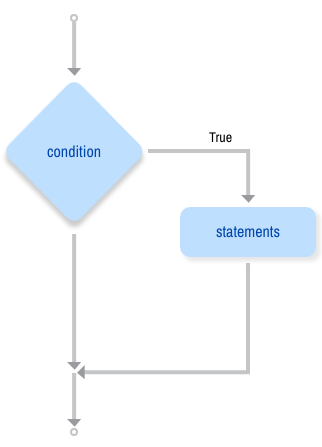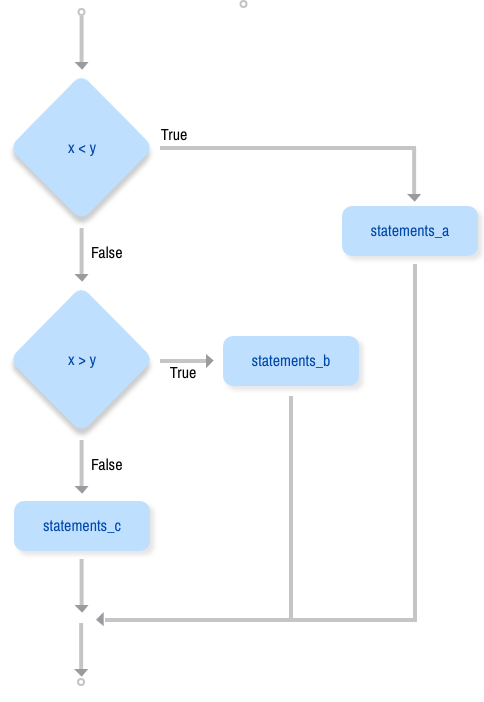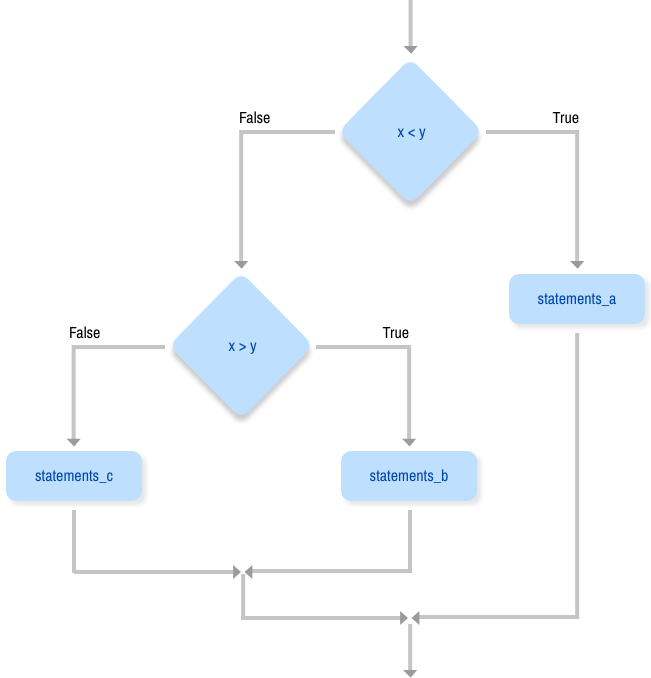# Python 3 Conditional Statements: If, If Else and Nested If Statements

In a Python program, the `if` statement is how you perform this sort of decision-making. It allows for conditional execution of a statement or group of statements based on the value of an expression.

The outline of this tutorial is as follows:

• First, you'll get a quick overview of the `if` statement in its simplest form.
• Next, using the `if` statement as a model, you'll see why control structures require some mechanism for grouping statements together into compound statements or blocks. You'll learn how this is done in Python.
• Lastly, you'll tie it all together and learn how to write complex decision-making code.

## The `if` Statement

We'll start by looking at the most basic type of `if` statement. In its simplest form, it looks like this:

``````if <expr>:
<statement>``````

In the form shown above:

• `<expr>` is an expression evaluated in Boolean context, as discussed in the section on Logical Operators in the Operators and Expressions in Python tutorial.
• `<statement>` is a valid Python statement, which must be indented. (You will see why very soon.)

If `<expr>` is true (evaluates to a value that is "truthy"), then `<statement>` is executed. If `<expr>`is false, then `<statement>` is skipped over and not executed.

Note that the colon (`:`) following `<expr>` is required. Some programming languages require `<expr>` to be enclosed in parentheses, but Python does not.

Here is the Flowchart of an if statement:Here are several examples of this type of `if` statement:

``````>>> x = 0
>>> y = 5

>>> if x < y:                            # Truthy
...     print('yes')
...
yes
>>> if y < x:                            # Falsy
...     print('yes')
...

>>> if x:                                # Falsy
...     print('yes')
...
>>> if y:                                # Truthy
...     print('yes')
...
yes

>>> if x or y:                           # Truthy
...     print('yes')
...
yes
>>> if x and y:                          # Falsy
...     print('yes')
...

>>> if 'aul' in 'grault':                # Truthy
...     print('yes')
...
yes
>>> if 'quux' in ['foo', 'bar', 'baz']:  # Falsy
...     print('yes')
...``````

Note: If you are trying these examples interactively in a REPL session, you'll find that, when you hit Enter after typing in the `print('yes')` statement, nothing happens.

Because this is a multiline statement, you need to hit Enter a second time to tell the interpreter that you're finished with it. This extra newline is not necessary in code executed from a script file.

## The `if else` statement

It is frequently the case that you want one thing to happen when a condition it true, and something else to happen when it is false. For that we have the `if else` statement.

``````if food == 'spam':
print('Ummmm, my favorite!')
else:
print("No, I won't have it. I want spam!")``````

Here, the first print statement will execute if `food` is equal to `'spam'`, and the print statement indented under the `else` clause will get executed when it is not.

Here is the Flowchart of a if else statementThe syntax for an `if else` statement looks like this:

``````if BOOLEAN EXPRESSION:
STATEMENTS_1        # executed if condition evaluates to True
else:
STATEMENTS_2        # executed if condition evaluates to False``````

Each statement inside the `if` block of an `if else` statement is executed in order if the boolean expression evaluates to `True`. The entire block of statements is skipped if the boolean expression evaluates to `False`, and instead all the statements under the `else` clause are executed.

There is no limit on the number of statements that can appear under the two clauses of an `if else` statement, but there has to be at least one statement in each block. Occasionally, it is useful to have a section with no statements (usually as a place keeper, or scaffolding, for code you haven’t written yet). In that case, you can use the `pass`statement, which does nothing except act as a placeholder.

``````if True:          # This is always true
pass          # so this is always executed, but it does nothing
else:
pass``````

## The `else` and `elif` Clauses

Sometimes there are more than two possibilities and we need more than two branches. One way to express a computation like that is a chained conditional:

``````if x < y:
STATEMENTS_A
elif x > y:
STATEMENTS_B
else:
STATEMENTS_C``````

Here is the Flowchart of chained conditional`elif` is an abbreviation of `else if`. Again, exactly one branch will be executed. There is no limit of the number of `elif` statements but only a single (and optional) final `else` statement is allowed and it must be the last branch in the statement:

``````if choice == 'a':
print("You chose 'a'.")
elif choice == 'b':
print("You chose 'b'.")
elif choice == 'c':
print("You chose 'c'.")
else:
print("Invalid choice.")``````

Each condition is checked in order. If the first is false, the next is checked, and so on. If one of them is true, the corresponding branch executes, and the statement ends. Even if more than one condition is true, only the first true branch executes.

## Nested Conditionals

One conditional can also be nested within another. (It is the same theme of composibility, again!) We could have written the previous example as follows:

``````if x < y:
STATEMENTS_A
else:
if x > y:
STATEMENTS_B
else:
STATEMENTS_C``````

Here is the Flowchart of this nested conditional:The outer conditional contains two branches. The second branch contains another `if` statement, which has two branches of its own. Those two branches could contain conditional statements as well.

Although the indentation of the statements makes the structure apparent, nested conditionals very quickly become difficult to read. In general, it is a good idea to avoid them when you can.

Logical operators often provide a way to simplify nested conditional statements. For example, we can rewrite the following code using a single conditional:

``````if 0 < x:            # assume x is an int here
if x < 10:
print("x is a positive single digit.")``````

The `print` function is called only if we make it past both the conditionals, so we can use the `and` operator:

``````if 0 < x and x < 10:
print("x is a positive single digit.")``````

Note: Python actually allows a short hand form for this, so the following will also work:

``````if 0 < x < 10:
print("x is a positive single digit.")``````

## One-Line `if` Statements

It is customary to write `if <expr>` on one line and `<statement>` indented on the following line like this:

``````if <expr>:
<statement>``````

But it is permissible to write an entire `if` statement on one line. The following is functionally equivalent to the example above:

``if <expr>: <statement>``

There can even be more than one `<statement>` on the same line, separated by semicolons:

``if <expr>: <statement_1>; <statement_2>; ...; <statement_n>``

But what does this mean? There are two possible interpretations:

1. If `<expr>` is true, execute `<statement_1>`.

Then, execute `<statement_2> ... <statement_n>` unconditionally, irrespective of whether `<expr>` is true or not.

2. If `<expr>` is true, execute all of `<statement_1> ... <statement_n>`. Otherwise, don’t execute any of them.

Python takes the latter interpretation. The semicolon separating the `<statements>` has higher precedence than the colon following `<expr>`—in computer lingo, the semicolon is said to bind more tightly than the colon. Thus, the `<statements>` are treated as a suite, and either all of them are executed, or none of them are:

``````>>> if 'f' in 'foo': print('1'); print('2'); print('3')
...
1
2
3
>>> if 'z' in 'foo': print('1'); print('2'); print('3')
...``````

Multiple statements may be specified on the same line as an `elif` or `else` clause as well:

``````>>> x = 2
>>> if x == 1: print('foo'); print('bar'); print('baz')
... elif x == 2: print('qux'); print('quux')
... else: print('corge'); print('grault')
...
qux
quux

>>> x = 3
>>> if x == 1: print('foo'); print('bar'); print('baz')
... elif x == 2: print('qux'); print('quux')
... else: print('corge'); print('grault')
...
corge
grault``````

While all of this works, and the interpreter allows it, it is generally discouraged on the grounds that it leads to poor readability, particularly for complex `if` statements. PEP 8specifically recommends against it.

As usual, it is somewhat a matter of taste. Most people would find the following more visually appealing and easier to understand at first glance than the example above:

``````>>> x = 3
>>> if x == 1:
...     print('foo')
...     print('bar')
...     print('baz')
... elif x == 2:
...     print('qux')
...     print('quux')
... else:
...     print('corge')
...     print('grault')
...
corge
grault``````

If an `if` statement is simple enough, though, putting it all on one line may be reasonable. Something like this probably wouldn’t raise anyone’s hackles too much:

``````debugging = True  # Set to True to turn debugging on.

.
.
.

if debugging: print('About to call function foo()')
foo()``````

## The `pass` Statement

Occasionally, you may find that you want to write what is called a code stub: a placeholder for where you will eventually put a block of code that you haven’t implemented yet.

In languages where token delimiters are used to define blocks, like the curly braces in Perl and C, empty delimiters can be used to define a code stub. For example, the following is legitimate Perl or C code:

``````# This is not Python
if (x)
{
}``````

Here, the empty curly braces define an empty block. Perl or C will evaluate the expression `x`, and then even if it is true, quietly do nothing.

Because Python uses indentation instead of delimiters, it is not possible to specify an empty block. If you introduce an `if` statement with `if <expr>:`, something has to come after it, either on the same line or indented on the following line.

Consider this script `foo.py`:

``````if True:

print('foo')``````

If you try to run `foo.py`, you’ll get this:

``````C:\Users\john\Documents\Python\doc>python foo.py
File "foo.py", line 3
print('foo')
^
IndentationError: expected an indented block``````

The `pass` statement solves this problem in Python. It doesn’t change program behavior at all. It is used as a placeholder to keep the interpreter happy in any situation where a statement is syntactically required, but you don’t really want to do anything:

``````if True:
pass

print('foo')``````

Now `foo.py` runs without error:

``````C:\Users\john\Documents\Python\doc>python foo.py
foo``````

## Conclusion

By using conditional statements like the `if` statement, you will have greater control over what your program executes. Conditional statements tell the program to evaluate whether a certain condition is being met. If the condition is met it will execute specific code, but if it is not met the program will continue to move down to other code.

temp